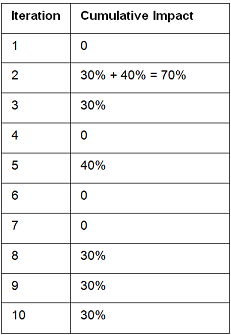Search Help:

﻿

About Project Risk Scores and Rankings

Project risk scores represent the level of risk in the project. The project risk score allows you to compare and rank projects based on their risk exposure. Project risk scores are shown in the Portfolio Gantt and Project Portfolio views. RiskyProject calculates three risk scores separately:

• For duration
• For cost
• For non-schedule risk categories

How Cost and Schedule Risk Scores Are Calculated

Project duration score is calculated using on the following formula:Where:

Risked Duration – could be mean project duration as a result of analysis, mean project duration plus a standard deviation of duration, or a certain percentile of the entire project duration.

Original Duration- Original (baseline) project duration with no risks and uncertainties

• If the project does not have duration risks, the Duration score will be equal 1.
• If the Duration risk score is >1, the project has threats related to duration.
• If the Duration risk score is <1,the project has opportunities related to duration.

How Non-Schedule and Cost Risk Scores Are Calculated

Risk scores for non-schedule or cost risks are calculated in the following manner:

1. For each project and category there is an array for risk results. For example, if there are two risks “Quality of installation” (probability 50%, impact 30%) and “Quality of manufacturing” (probability 20%, impact 40%), the array for the quality category may look like this:
2.3. The mean of this array is calculated and multiplied by the weight for each non-schedule risk category
4. The sum of values from Step 2 is calculated for the particular project for all risk categories; this number represents the absolute non-schedule risk score for the particular project.
5. Absolute non-schedule risk scores for all projects are normalized in such a way that:
• if a project does not have any risks, the score will = 1
• if a project has opportunities, the score will be < 1
• if a project has threats, the score will be > 1.

In this way the risk scores for non-schedule risks will be compatible with risk scores for duration and cost.